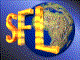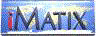| iMatix home page | << | < | > | >>SFLVersion 2.11

### set_curdir

```#include "sfldir.h"
int
set_curdir (
const char *path)
```

#### Synopsis

Sets the current working directory as specified. Returns 0 if the directory path was found; -1 if there was an error. Under Windows, replaces '/' by '\' before changing directory, and switches to the specified disk if the path starts with a letter and ':'. Does nothing if the path is NULL or empty.

#### Source Code - (sfldir.c)

```{
int
feedback = 0;

#if (defined (__UNIX__) || defined (__VMS_XOPEN) || defined (__OS2__))
if (path && *path)
feedback = chdir (path);

#elif (defined (MSDOS_FILESYSTEM))
char
*copy_path = mem_strdup (path);

if (path == NULL || *path == '\0')
return (0);                     /*  Do nothing if path is empty      */

/*  MS-DOS compilers generally require a two-step process                */
strconvch (copy_path, '/', '\\');

#   if (defined (WIN32))
/* The drive letter does not need to be changed separately in Win32.    */
feedback = !SetCurrentDirectory (copy_path);

#   elif (defined (__TURBOC__))
feedback = chdir (copy_path);
if (feedback == 0 && isalpha (path ) && path  == ':')
setdisk (toupper (path ) - 'A');

#   elif (defined (__LCC__))
feedback = chdir (copy_path);
if (feedback == 0 && isalpha (path ) && path  == ':')
chdrive (toupper (path ) - 'A' + 1);

#   elif (defined (_MSC_VER))
feedback = _chdir (copy_path);
if (feedback == 0 && isalpha (path ) && path  == ':')
_chdrive (toupper (path ) - 'A' + 1);

#   endif
mem_strfree (&copy_path);
#else
feedback = -1;
#endif

return (feedback);
}
```

 | << | < | > | >>Copyright © 1996-2000 iMatix Corporation